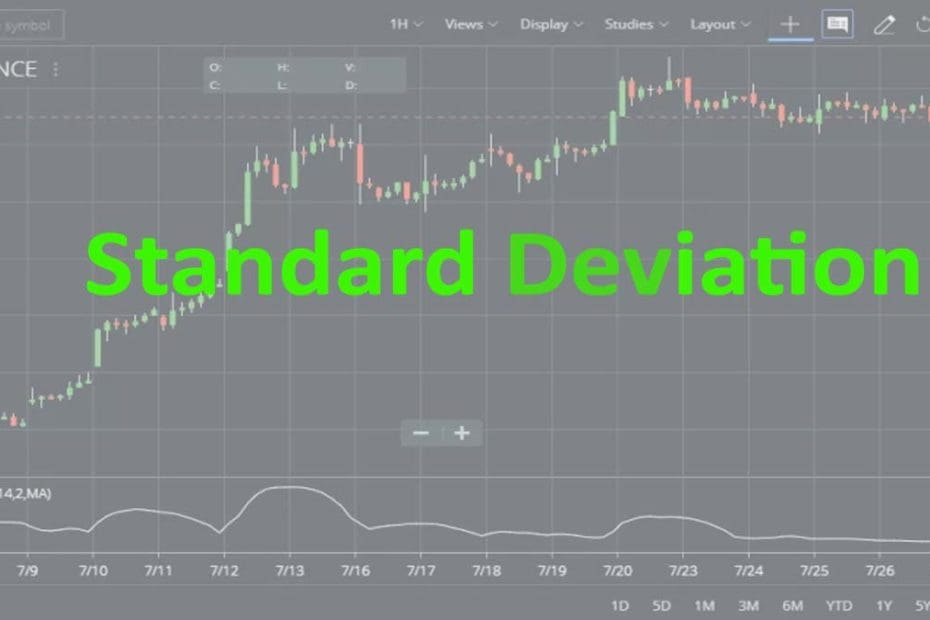Home » Blog » Trading » Technical Indicators » Standard Deviation Indicator Strategy, Formula, Definition

# Standard Deviation Indicator Strategy, Formula, Definition

• Technical IndicatorsStandard deviation is a statistical term that measures the amount of variability or dispersion around an average. It is also a measure of volatility. Standard deviation Indicator dispersion is the difference between the actual value and the average value. This article will explain the Standard Deviation Indicator.

## What is a Standard Deviation Indicator?

This indicator describes the range of price fluctuations relative to the Moving Average. This indicator is mainly used in technical analysis and trading systems to statistically measure a stock’s volatility by showing the difference between the price and the average price. Generally, this indicator is used as a component of other indicators.

## What is the usage of the Standard Deviation Indicator?

• The most common usage of the standard deviation indicator is to forecast price reversals based on the principle of reversion to the mean.
• Traders take Standard deviation as one of the most reliable indicators available to traders but under specific conditions.
• In the trending markets, the volatility is moderate and price oscillation is concentrated around the middle range. The SD indicator is one of the best popular tools one could find.

## How to Set up the Standard Deviation Indicator on your Charts?

If traders want to know more about the Standard Deviation Indicator, then they can find the same in the STUDIES section of Zerodha Kite. Kite mobile App to has this indicator incorporated in it. The default Period is 14 and you can set the value of it high and low. The Field is Close you can change this. The Standard Deviations is 2 and you can change this value high or low. The Moving Average Type of indicator is simple. In this indicator on to any charts likes daily, weekly, monthly or intraday you can attach. You can also check how we attached the Indicator in Reliance Industries share price chart.

The next charting platform I am going to explain is Upstox pro. So, like Zerodha kite, open your Upstox account. Then open a chart and the indicator section. Type down the indicator name and click on apply.

## Calculation of Standard Deviation Indicator :

Standard deviation (SD) = Sqrt [(Sum the ((Close for each of the past n Periods – n Period SMA for the current bar)^2))/ n].

## How this indicator works :

• Standard deviation rises as prices become more volatile. As price action calms, standard deviation heads lower.
• Standard deviation is increased with moving price and it shows above-average strength or weakness.
• In this indicator market tops are accompanied by increased volatility over short periods of time indicate nervous and indecisive traders. Also, market tops with decreasing volatility over long time frames indicate maturing bull markets.
• In the indicator market, bottoms that are accompanied by decreased volatility over long periods of time indicate bored and disinterested traders. Also, market bottoms with increasing volatility over relatively short time periods indicate panic sell-offs.

## What are the Trading Strategies of the Standard Deviation?

• If uptrend and Standard Deviation crosses its middle line at a price reduction (correction in a bullish trend) − we BUY;
• if the trend is downward and indicator pierces the average with an increase in price (correction within the bearish trend) − we SELL.
• Indicator can be used as a trend filter in combination with oscillators − in case of breakdown of the Standard Deviation line, a transaction is made in direction of the trend. An example is the SD+RSI system, where the trend indicator is StDev, and the signal for the transaction is RSI indicators in direction of Standard Deviation.

## Conclusion :

The standard deviation is a statistical measure of volatility. In this indicator, the price moves greater than the standard deviation and show it above average strength or weakness. It is also used with other indicators like Bollinger Bands. In this indicator moves that exceed the bands are deemed significant enough to warrant attention. The indicator should be used in conjunction with other analysis tools as with all indicators.